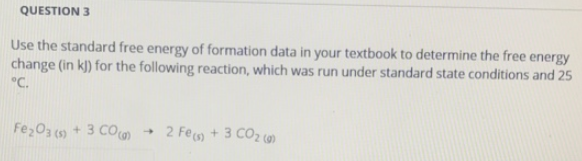# Problem: Use the standard free energy of formation data in your textbook to determine the free energy change (in kJ) for the following reaction, which was run under standard state conditions and 25 °C. Fe2O3 (s) + 3CO (g) → 2Fe (s) + 3CO2 (g)

###### FREE Expert Solution
84% (349 ratings)###### Problem Details

Use the standard free energy of formation data in your textbook to determine the free energy change (in kJ) for the following reaction, which was run under standard state conditions and 25 °C.

Fe2O(s) + 3CO (g) → 2Fe (s) + 3CO2 (g)